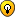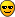## ALTERNATIVE IMAGE FORMAT

NTech
Posts: 33
Joined: Mon May 01, 2017 7:29 pm
Location: USA

### ALTERNATIVE IMAGE FORMAT

The winning entry will make it possible to draw an image, and save
it in a format, like this:

1 1,200 200 200 //THIS IS THE PIXEL'S X AND Y AND THE PIXELS COLOR.
1 2,200 200 200 //AND SOFORTH.

The 1 and 2 mean the x and y. The 200 200 200 represent the pixels color.
The file is basically the x,y,and pixelColor. A new line of code is
what is the use of a new file you cant acess? I would reccomend using
the getPixelValue function included in the risk game. Also, i would
use the functions by uncleBen in his sprite-creator, to get the bmp's
width and height. Please post if there is anybody who is interested.
Other then that, i am working on my version, but that is 0% complete.
Get to it!NTech
Posts: 33
Joined: Mon May 01, 2017 7:29 pm
Location: USA

### Re: ALTERNATIVE IMAGE FORMAT

I have made something like this, though I tweaked my original idea. The result is a new image format, slightly larger than bitmaps.
Try it out. Be warned - conversion to BPG image type takes a while, so try small images until you get used to it. Fortunately, rendering a BPG is much faster...Code: Select all

``````'BPG - Image Format Prototype
'--Files are a little bit larger than bitmap images, though not by much.

dim b\$(26)
b\$(1) = "b"
b\$(2) = "c"
b\$(3) = "d"
b\$(4) = "e"
b\$(5) = "f"
b\$(6) = "g"
b\$(7) = "h"
b\$(8) = "i"
b\$(9) = "j"
b\$(10) = "k"
b\$(11) = "l"
b\$(12) = "m"
b\$(13) = "n"
b\$(14) = "o"
b\$(15) = "p"
b\$(16) = "q"
b\$(17) = "r"
b\$(18) = "s"
b\$(19) = "t"
b\$(20) = "u"
b\$(21) = "v"
b\$(22) = "w"
b\$(23) = "x"
b\$(24) = "y"
b\$(25) = "z"
b\$(26) = "1"

nomainwin

WindowWidth = 550
WindowHeight = 410

open "BPG Main" for graphics_nsb_nf as #main
print #main, "font ms_sans_serif 0 16;flush seg"

#main, "fill green;getbmp g 0 0 1 1;fill white;delsegment seg;flush seg;trapclose [quit]"

'a\$ = GetPixelValue\$(1,1)

'notice GetPixelValue

wait

[quit]
#main, "delsegment seg"
if load = 1 then close #b
close #main
end

filedialog "Select A Bitmap Image", "*.*", file\$
if file\$ = "" then wait

prompt "width";width
prompt "height";height

#main, "drawbmp img 0 0;delsegment seg;flush seg"

wait

[convert]
frame = 0
filedialog "Save As", "*.bpg", file\$
if file\$ = "" then wait
open file\$ for output as #b
#b, height;" ";width
wi = width
he = height
for q = 1 to wi
for w = 1 to he
scan
#main, "getbmp v "; q; " "; w; " "; 1; " "; 1
bmpsave "v", "t.t"
open "t.t" for input as #v
s\$ = input\$(#v, lof(#v))
close #v
'if asc(mid\$(s\$, 29, 1)) = 32 then
r = asc(mid\$(s\$, 69, 1))
g = asc(mid\$(s\$, 68, 1))
b = asc(mid\$(s\$, 67, 1))
'end if

#main, "drawbmp g ";q;" ";w
#b, roundT\$(r);roundT\$(g);roundT\$(b)
next w
next q
close #b

'notice "Complete"
goto [quit]

wait

filedialog "Select A Bpg Image", "*.bpg", file\$
if file\$ = "" then wait

open file\$ for input as #b
input #b, lir\$
height = val(word\$(lir\$,1))
width = val(word\$(lir\$,2))
for q = 1 to width
for w = 1 to height
scan
input #b, lin\$
#main, "color ";unRoundT(left\$(lin\$,1));" ";unRoundT(right\$(left\$(lin\$,2),1));" ";unRoundT(right\$(left\$(lin\$,3),1));";down;set ";q;" ";w;";up"
next w
next q
close #b
#main, "delsegment seg;flush seg"
wait

[save]
filedialog "Save As", "*.bmp", file\$
if file\$ = "" then wait

#main, "getbmp bit 0 0 ";width;" ";height
bmpsave "bit", file\$

wait

[notes]
notice "BPG NOTES";chr\$(13);"This compiles and displays BPG format.";chr\$(13);"The compilation is much longer than the rendering.";chr\$(13);"All Rights Reserved 2018, Ntech"
wait

function GetPixelValue\$(x,y)
#main, "getbmp gpv "; x; " "; y; " "; 1; " "; 1
bmpsave "gpv", "t.t"
open "t.t" for input as #gpv
s\$ = input\$(#gpv, lof(#gpv))
close #gpv
GetPixelValue = (red + green + blue)
end function

function roundT\$(num)
if num < 10 then
roundT\$ = "a"
exit function
end if

for i = 1 to 26
if num = (i*10) then
print i*10
roundT\$ = b\$(i)
end if
if num > (i*10) and num < ((i+1)*10) then
print i*10
roundT\$ = b\$(i)
exit for
end if
next i
end function

function unRoundT(num\$)
if num\$ = "a" then
unRoundT = 5
exit function
end if

for i = 1 to 26
if num\$ = b\$(i) then
unRoundT = (i*10)
exit for
end if
next i
end function
``````
I will make adjustments to the code, and maybe work on compression. Until then, here it is!